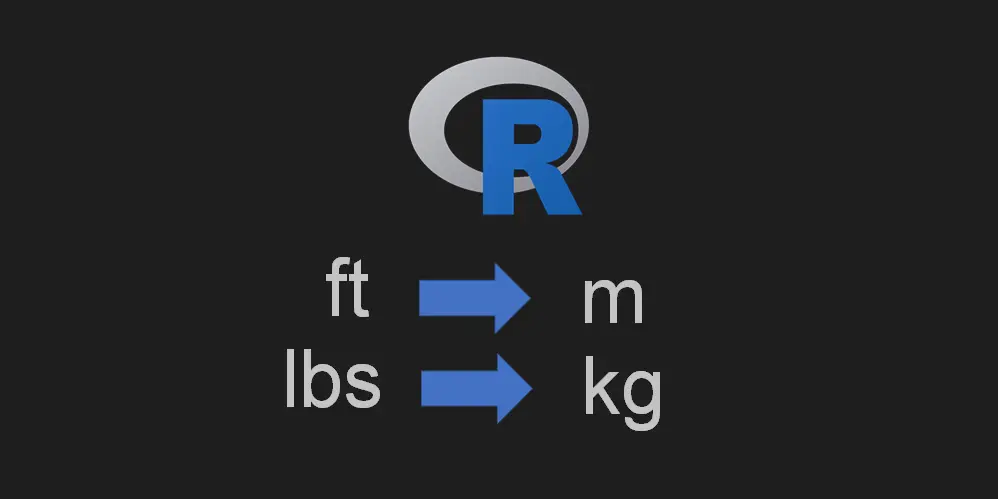# How to convert units in R like ft to m or lbs to kg

Here is a simple solution that helps to convert units in R and, in this case, feet to meters and pounds to kilograms. It is not always straightforward, and you have to do additional data transformations to get to the point where you can do the unit conversion in R.

Here is a dataset that contains information about NBA players. I already selected a unique list of them and only columns that contain their name, height in feet, and weight in pounds.

```nba <- NBAloveR::players

require(dplyr)

nba <- nba %>% select(Player, HT, WT) %>% distinct()

#              Player   HT  WT
#1     John Abramovic  6-3 195
#2      Chet Aubuchon 5-10 137
#3         Norm Baker  6-0 180
#4 Herschel Baltimore  6-4 195
#5          John Barr  6-3 205
#6  Frankie Baumholtz 5-10 170```

First of all, I would like to convert feet to meters by using column HT, but at this point, it is not numerical.

```class(nba\$HT)

# "factor"
```

In this case, I will replace necessary characters to get feet as a decimal number. Take a look at this post if you want to know how to replace special characters like a new line or tab.

```nba\$HT <- as.numeric(gsub("-", ".", nba\$HT))

#              Player  HT  WT
#1     John Abramovic 6.3 195
#2      Chet Aubuchon 5.1 137
#3         Norm Baker 6.0 180
#4 Herschel Baltimore 6.4 195
#5          John Barr 6.3 205
#6  Frankie Baumholtz 5.1 170```

As you can see, now it looks like something that you can convert. It is important that the column is numerical. There is also a problem with the column that contains weight data because it is a factor.

```class(nba\$WT)

# "factor"```

Here is how to convert factors to numerical.

`nba\$WT <- as.numeric(as.character(nba\$WT))`

## Convert units in R

To convert ft to m by using R, I will use the conv_unit function from the measurements package. Simple conversions like from seconds to minutes you can do mathematically.

You can do a wide variety of conversions with this function, but feet to meters in R looks like this.

```nba\$HT_in_m <- measurements::conv_unit(nba\$HT, "ft", "m")

#              Player  HT  WT HT_in_m
#1     John Abramovic 6.3 195 1.92024
#2      Chet Aubuchon 5.1 137 1.55448
#3         Norm Baker 6.0 180 1.82880
#4 Herschel Baltimore 6.4 195 1.95072
#5          John Barr 6.3 205 1.92024
#6  Frankie Baumholtz 5.1 170 1.55448```

Here is how to convert lbs to kg.

```nba\$WT_in_kg <- measurements::conv_unit(nba\$WT, "lbs", "kg")

#              Player  HT  WT HT_in_m WT_in_kg
#1     John Abramovic 6.3 195 1.92024 88.45052
#2      Chet Aubuchon 5.1 137 1.55448 62.14216
#3         Norm Baker 6.0 180 1.82880 81.64664
#4 Herschel Baltimore 6.4 195 1.95072 88.45052
#5          John Barr 6.3 205 1.92024 92.98645
#6  Frankie Baumholtz 5.1 170 1.55448 77.11071```

As you can see, the result contains many decimal places. I will round the results to keep only two of them.

```nba\$HT_in_m <- round(nba\$HT_in_m, digits = 2)

nba\$WT_in_kg <- round(nba\$WT_in_kg, digits = 2)

#              Player  HT  WT HT_in_m WT_in_kg
#1     John Abramovic 6.3 195    1.92    88.45
#2      Chet Aubuchon 5.1 137    1.55    62.14
#3         Norm Baker 6.0 180    1.83    81.65
#4 Herschel Baltimore 6.4 195    1.95    88.45
#5          John Barr 6.3 205    1.92    92.99
#6  Frankie Baumholtz 5.1 170    1.55    77.11```

Here is a post about rounding in R. Keep in mind that the round function does the rounding to the even digit.

You can select necessary records and check the results, but that is highly dependent on the precision of the initial data.

```subset(nba, nba\$Player == "Kristaps Porzingis")

#                 Player  HT  WT HT_in_m WT_in_kg
#4039 Kristaps Porzingis 7.3 240    2.23   108.86```

If you would like to rearrange columns in the R data frame, then here is how to do that.

Categories R